# Geometry B 2016-2017 SSC (English Medium) 10th Standard Board Exam Question Paper Solution

Geometry [B]
Date & Time: 16th March 2017, 11:00 am
Duration: 2h

 1 | Solve any five sub-equations
 1.1

In the following figure, seg BE ⊥ seg AB and seg BA ⊥ seg AD. If BE = 6 and $\text{AD} = 9 \text{ find} \frac{A\left( \Delta ABE \right)}{A\left( \Delta BAD \right)} \cdot$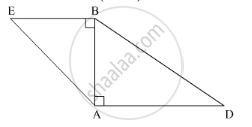Concept: Similarity of Triangles
Chapter: [0.01] Similarity
 1.2

If two circles with radii 8 cm and 3 cm, respectively, touch internally, then find the distance between their centers.

Concept: Touching Circles
Chapter: [0.03] Circle
 1.3

Find the height of an equilateral triangle whose side is 6 units.

Concept: 30 - 60 - 90 and 45 - 45 - 90 Theorem
Chapter: [0.02] Pythagoras Theorem
 1.4

If the angle θ = –45° , find the value of tan θ.

Concept: Trigonometric Ratios of Complementary Angles
Chapter: [0.06] Trigonometry
 1.5

Find the slope and y – intercept of the line y = 3x – 5.

Concept: Intercepts Made by a Line
Chapter: [0.04] Co-ordinate Geometry
 1.6

Find the circumferences of a circle whose radius is 7 cm.

Concept: Tangents and Its Properties
Chapter: [0.03] Circle
 2 | Solve any four sub-questions
 2.1

In the following figure, in ΔPQR, seg RS is the bisector of ∠PRQ. If PS = 6, SQ = 8, PR = 15, find QR.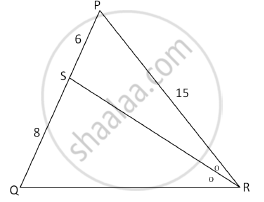Concept: Similarity of Triangles
Chapter: [0.01] Similarity
 2.2

In the given figure PA = 10, PB = 2 and PC = 5. Find PD.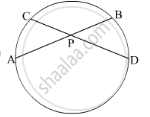Concept: Number of Tangents from a Point on a Circle
Chapter: [0.03] Circle [0.03] Circle
 2.3

Draw ∠ABC of measures 135°and bisect it.

Concept: Basic Geometric Constructions
Chapter: [0.05] Geometric Constructions
 2.4

Find the sine ratio of θ in standard position whose terminal arm passes through (3, 4)

Concept: Trigonometric Ratios of Complementary Angles
Chapter: [0.06] Trigonometry
 2.5

Find the slope of the line passing through the points G(4, 5) and H (–1, –2).

Concept: Slope of a Line
Chapter: [0.04] Co-ordinate Geometry
 2.6

The dimensions of a cuboid in cm are 50 × 18 × 10. Find its volume.

Concept: Surface Area of a Combination of Solids
Chapter: [0.07] Mensuration
 3 | Solve any three sub-questions ;
 3.1

Prove that: If the angles of a triangle are 45° – 45° – 90°, then each of the perpendicular sides is $\frac{1}{\sqrt{2}}$times the hypotenuse.”

Concept: Angles in Standard Position
Chapter: [0.06] Trigonometry
 3.2

Find the angle between two radii at the centre of the circle as shown in the figure. Lines PA and PB are tangents to the circle at other ends of the radii and ∠APR = 140°.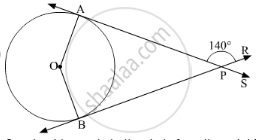Concept: Number of Tangents from a Point on a Circle
Chapter: [0.03] Circle [0.03] Circle
 3.3

Construct tangents to the circle from the point B, having radius 3.2 cm and centre 'C'. Point B is at a distance 7.6 cm from the centre.

Concept: Construction of Tangents to a Circle
Chapter: [0.05] Geometric Constructions
 3.4

From the top of a lighthouse, an observer looks at a ship and finds the angle of depression to be 60° . If the height of the lighthouse is 90 meters, then find how far is that ship from the lighthouse? (√3 = 1.73)

Concept: Heights and Distances
Chapter: [0.06] Trigonometry
 3.5

The volume of a cube is 343 cm3. Find its total surface area.

Concept: Surface Area of a Combination of Solids
Chapter: [0.07] Mensuration
 4 | Solve any two sub questions :
 4.1

Prove that “The opposite angles of a cyclic quadrilateral are supplementary”.

Concept: Cyclic Properties
Chapter: [0.03] Circle
 4.2

Eliminate θ, if
x = 3 cosec θ + 4 cot θ
y = 4 cosec θ – 3 cot θ

Concept: Trigonometric Identities
Chapter: [0.06] Trigonometry
 4.3

A toy is a combination of a cylinder, hemisphere and a cone, each with radius 10 cm as shown in the figure. Height of the conical part is 10 cm and total height is 60 cm. Find the total surface area of the toy.
(π=3.14, √2=1.41)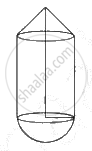Concept: Surface Area of a Combination of Solids
Chapter: [0.07] Mensuration
 5 | Solve any two sub–questions;
 5.1

In the given figure, AD is the bisector of the exterior ∠A of ∆ABC. Seg AD intersects the side BC produced in D. Prove that :

$\frac{BD}{CD} = \frac{AB}{AC}$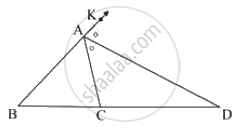Concept: Properties of Ratios of Areas of Two Triangles
Chapter: [0.01] Similarity
 5.2

Construct the circumcircle and incircle of an equilateral ∆XYZ with side 6.5 cm and centre O. Find the ratio of the radii of incircle and circumcircle.

Concept: Division of a Line Segment
Chapter: [0.04] Co-ordinate Geometry [0.05] Geometric Constructions
 5.3

A (5, 4), B (–3,–2) and C (1,–8) are the vertices of a triangle ABC. Find the equation of median AD and line parallel to AB passing through point C.

Concept: General Equation of a Line
Chapter: [0.04] Co-ordinate Geometry

#### Request Question Paper

If you dont find a question paper, kindly write to us

View All Requests

#### Submit Question Paper

Help us maintain new question papers on Shaalaa.com, so we can continue to help students

only jpg, png and pdf files

## Maharashtra State Board previous year question papers 10th Standard Board Exam Geometry with solutions 2016 - 2017

Maharashtra State Board 10th Standard Board Exam Geometry question paper solution is key to score more marks in final exams. Students who have used our past year paper solution have significantly improved in speed and boosted their confidence to solve any question in the examination. Our Maharashtra State Board 10th Standard Board Exam Geometry question paper 2017 serve as a catalyst to prepare for your Geometry board examination.
Previous year Question paper for Maharashtra State Board 10th Standard Board Exam Geometry-2017 is solved by experts. Solved question papers gives you the chance to check yourself after your mock test.
By referring the question paper Solutions for Geometry, you can scale your preparation level and work on your weak areas. It will also help the candidates in developing the time-management skills. Practice makes perfect, and there is no better way to practice than to attempt previous year question paper solutions of Maharashtra State Board 10th Standard Board Exam.

How Maharashtra State Board 10th Standard Board Exam Question Paper solutions Help Students ?
• Question paper solutions for Geometry will helps students to prepare for exam.
• Question paper with answer will boost students confidence in exam time and also give you an idea About the important questions and topics to be prepared for the board exam.
• For finding solution of question papers no need to refer so multiple sources like textbook or guides.